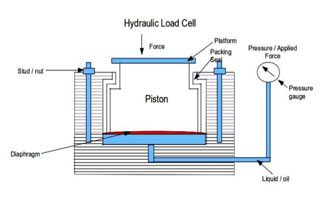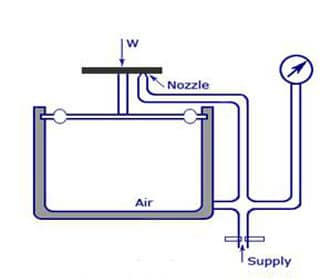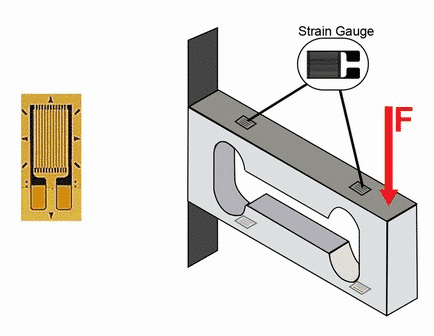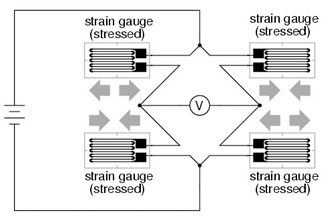# Load Cell Weight

### CAN YOU USE A SINGLE LOAD CELL TO MEASURE CONSTANT WEIGHT?

A load cell is an instrument that helps to determine the size of a load (either a force or a weight) and can also give the direction to which the load is acting.

Technically, a load cell is a sensor that detects changes in a physical stimulus (in this case, a force, weight or pressure) and then produces an output proportional to the physical stimulus. Therefore, for a certain constant load or weight size, the load cell gives an output value that is proportional to the magnitude of the weight.

## Types of Load Cells

In order to measure a constant weight or any loads size using a single load cell, there are wide varieties of options available. There are different types of load cells for weight measurement, each for various applications.

The most commonly used types are:

• Strain gauge load cell
• Capacitance load cell
• Hydraulic load cell
• Pneumatic load cell

The first two types (strain gauge and capacitance load cell) are electrical transducer devices. An electrical transducer is a sensor that detects a physical stimulus and then produces an electrical analog signal (voltage) as its output. The other two devices (hydraulic and pneumatic load cell) do not directly produce output as electrical signals but they can have their output transduced by other means depending on the application requirement.

The most widely used type among all load cell types is the strain gauge load cell. This device can be found in all industries such as medicine, automation, automotive, domestic etc. hence, to measure a constant weight using a load cell, you will have a wide variety of strain gauge types to choose from. The advantages of this type will be seen in the latter sections of the article.

## The Design and the Mode of Operation of the Load Cells

The decision to use a single load cell to measure a constant weight over a period of time will require that the operator or user understands a little bit of information and details on how these stuff work.

Understanding the design and the mode of operation of these load cells will influence the decision on which type of load cell fits your requirements for determining the size of a certain weight.

### 1. Hydraulic Load Cell

• This type of load cell is a force-balance device. Its components are housed in a cylindrical frame made of stainless steel. Design The figure below shows a general arrangement of a hydraulic load cell. The device consists of the following parts: Figure 1. The Hydraulic load cellFigure 1. The Hydraulic Load Cell
• A Diaphragm: This is an elastic material or in the form of a corrugated plate.
• The Loading Surface or Platform: This is usually a piston that is designed as a loading platform to which the diaphragm is bonded. The diaphragm is attached to the base of this piston to make contact with the hydraulic fluid.
• The Hydraulic Fluid: The device is filled with oil or at times water.
• A Pressure Output: This might be a pressure gauge that is connected to the load cell through a bourdon tube. The Mode of Operation To measure a weight with this device, the weight is placed on the loading platform. The oil-filled chamber is connected to a pressure gauge and sealed by the diaphragm, hence, this applied weight will produce pressure increases in the confined oil. This is then indicated on the pressure gauge. As mentioned earlier, if you wish to obtain the output in the form of an electrical analog form, then the output can be transduced using a pressure transducer in place of the pressure gauge. It should be noted that the hydraulic load cells are stiff with a force range of up to 5MN and a system accuracy in the order of 0.25 to 1.0 percent.

## 2. The Pneumatic Load CellDesign

• The device consists of the following parts that are similar to those of the hydraulic type. The figure below shows a general arrangement of a pneumatic load cell.Figure 2. A Pneumatic Load Cell
• The Loading Platform: This is the surface on which the weight to be measured is placed
• An Elastic Diaphragm: It is elastic in nature and made up of a flexible material or can be made corrugated
• The Air Supply Regulator: This part is always located below the diaphragm or at the bottom of the load cell. This air supply regulator is simply an opening that is regulated by a valve.
• An Outlet Nozzle: This is an opening that does not have a direct valve that regulates it.
• The Flapper: This is also called the bleed valve. It is attached to the bottom of the loading platform together with the diaphragm. Its movement closes or opens the outlet nozzle.
• A Pressure Output(Manometer): This indicates the pressure as a measure of the weight or applied force. The Mode Operation The weight to be measured is placed on the loading platform. This then goes to exert a certain amount of pressure on the air inside the confined space as the diaphragm deflects. The deflection of the diaphragm and the increased internal air pressure causes the flapper to move downwards and shut off the outlet nozzle. This then balances the internal pressure and the resultant pressure causes the deflection of the dial in the pressure gauge. This amount then equals a value that is proportional to the actual weight size. This proportional constant is easily obtained by initially calibrating the load cell using a known weight. To return the diaphragm to its pre-loaded position, the air supply regulator is used, this device can hold load up to 25KN. A pressure transducer can also be fixed at the output so as to obtain an electrical analog output signal.

### 3. Capacitive Load CellDesign

• Capacitive Load Cell Design The basic design of a capacitive transducer is shown in the figure below
• A Stationary Plate: This is one of the two parallel plates and it is attached to the body of the insulated housing.
• A Moveable Plate: This is the second of the two parallel plates and it changes position under the influence of the weight. So the conventional design has a loading platform that is attached directly to this movable plate.
• The Dielectric: This is situated between the two parallel metallic plates. It is often made to be air, but can also be other elements or materials.
• The Insulated Housing: This provides the support and protection for the parts of the capacitive load cell The Mode of Operation The construction of the devices ensures that the two parallel metal plates are separated by a distance filled with a dielectric. A load or weight to be measured is then applied to the movable plate which deflects under this influence. This deflection then decrease the distance between the two plates, while the area of the plates and the dielectric remains constant The relationship between the device’s capacitance, the distance between its plates, the area, and the dielectric can be further understood by this mathematical formula below. Where C is the capacitance, is the relative permittivity, is the absolute permittivity, A is the cross-sectional area of the capacitive plates and d is the distance between the plates. This change in distance causes a change in capacitance, this can then be measured by using a Wheatstone bridge (Wien Bridge) or through the resonant circuit of an oscillator. The capacitance causes a voltage to be stored in the capacitor’s electric field, this voltage can also be amplified to indicate the capacitance of the device. Whichever method through which the capacitance is obtained, it shows the equivalent size of the weight that produced the capacitance. Therefore, a constant weight placed on a capacitive load cell will produce a constant value of capacitance. The value might change or vary within a certain range that depends on the accuracy of the device. Generally, capacitive load cells are very sensitive and they can measure static weight changes as well as dynamic changes. A resolution of about can be obtained.

### 4. Strain Gauge Load Cell Design

• The Elastic Element: : This is the part of the load cell that transduces the applied weight into a strain, in a proportion to the weight. Hence, it is called the primary transducer. This part makes up a large visible percentage of the load cell’s body and it is called the structural member. Generally, the geometric shape of this element determines the category to which the strain gauge load cell belongs. For example, a geometric shape in the form of a beam makes a beam strain gauge load cell. Furthermore, the geometric shape and the modulus of elasticity of this element govern the weight the lad cell can hold in a particular direction and the overall performance of the device.
• The Strain Gauge: This is a length of a conductor (metallic or semiconductor), which is first micro-machined on a polyester backing or a non-conductive layer to form a composite. The composite is then bonded to the elastic element in such a way that it receives a sufficient amount of the strain along the direction at which the weight is placed.Figure 3. The Strain gauge Load Cell.
• The strain gauge transduces the transduced strain into a proportional change in its electrical resistance. Hence, the strain gauge is the secondary transducer. The strain is directly proportional to the applied weight, hence, the change in the resistance is therefore equivalently proportional to the weight. This change in electrical resistance is then measured to indicate weight. So, when the strain gauge is subjected to a strain, its length, area and electrical resistance changes in accordance to this formula: Where R is the electrical resistance, is the resistivity of the gauge material, L is the length of the conductive strip, A is the cross-sectional area of the conductive strip. The strain gauge and the elastic element must be able to hold the constant weight for a period of time without breaking or exceeding their elastic limit. There are different types of strain gauges that offer unique characteristics and they include the wire strain gauge, the thin-film strain gauge, the foil strain gauge and the semiconductor strain gauge.
• The Housing Unit: This forms the protective casing of the whole load cell against unfavorable environmental conditions. It allows for external fixings such as mounting kits and screws to be attached to the load cell.

View These Types of Load Cells:

Double Ended Shear Beam

Disk Load Cells

S-Type / S-beam

The Mode of Operation

The weight to be measured is placed on the load application point of the load cell. The weight is transduced into strain by the elastic element, and the transduced strain is further transduced into a change in electrical resistance by the strain gauge as its dimensions changes under the influence of the strain caused by the weight.

The change is electrical resistance is then measured by using a Wheatstone bridge. If there are more than one strain gauges used, they are incorporated in a Wheatstone bridge circuit configuration to measure their collective change in resistance.

Therefore, it is the output of the Wheatstone bridge that forms the output of the strain gauge load cell. This output is in the form of an electrical analog voltage usually of the order of millivolts (mV).

A Wheatstone bridge has four arms, hence it can hold up to four strain gauges. The number of strain gauges on the arms of the bridge determines its configuration. A single strain gauge load cell will use the quarter-bridge configuration. A double strain gauge load cell will use the half-bridge configuration. A four strain gauge will use the full-bridge configuration.Figure 4. A Full-Bridge Configuration

The strain gauges acting the same direction (compression or tension) are placed in opposite arms of the bridge so as to maximize the response of the load cell.

Furthermore, to increase sensitivity (the rate of change of the output voltage with respect to the change in length) and also obtain accurate results, it is advised to get a load cell with more than one strain gauge.

## Calibration of the Load Cell

Calibration is the comparison of the reading of the instrument in question (the load cell to be used for measuring the constant weight) to a known standard and the maintenance of the evidentiary chain from that standard. Technically, it is called the Tractability.

The process of calibration helps to eliminate the errors that are inherent in the load cell. These errors are called the systematic errors. Calibration at sufficient intermediate points in the range of an instrument can help cover systematic non-linearity.

Also, microprocessor–based implementation of the whole measurement setup will reduce this same problem of systematic non-linearity. This is so because microprocessors can perform multipoint breakpoint linearization.

The load cell performance is influenced by other quantities such as temperature and even a change in the direction of measurement, hence constant calibration is needed. A formal calibration of the load cell [used for constant weight measurement] should be carried out at intervals of about 1-2 years to maintain accurate results.

Use: Calibration services at Tacuna Systems or check out our load cell calibration weights.

## Load Cell Specifications

The specifications of a load cell for weight measurement can easily be obtained from the manufacturer by asking for a datasheet. These specifications are of great importance when it comes to the accuracy of the load cell in providing a reliable result for a constant weight measured over a period of time.

These specifications include:

• The Zero Balance: This is the default output signal of the load cell when no weight is placed on it. When using a load cell, you can easily confirm this value by taking the output reading when all weights (including dead load) has been removed
• Non-Linearity: Normally, the output of the load cell is meant to be exactly proportional to the weight (at least form some intervals). However, in practical measurements, there are discrepancies and this called the non-linearity error. Non-linearity expresses the maximum deviation of the true input/output curve from the idealized straight line approximating it.
• Hysteresis: This is the difference between the outputs of a load cell as the weight is increased [from no load to the full rated capacity] and the output of a load cell as the weight is decreased [from the full rated capacity to no load]. For a constant weight application, this specification will not affect the result accuracy but it should still be taken into consideration.
• Non-Repeatability: This is the maximum difference between the outputs of the load cell for repeated loading under the same conditions. For a constant weight application, this should also not affect the accuracy of the measurement.
• Creep: This is the most important specification to pay attention to when it comes to a constant weight application. Creep depicts the change in the load cell output with time under a constant load and with all the environmental conditions being constant. This simply means that if you leave a constant weight on the load cell, the output reading changes by a certain percentage even though no weight is being added or removed.
• Temperature Effects on The Zero Balance and the Output: Temperature shifts affect the zero load position and the output of the load cell. Strain gauge load cells implore various effective temperature compensation techniques to cater for this effects.

## Conclusion

It can then be concluded that it is possible to use a single load cell to measure a constant weight over a long period of time. However, the accuracy of the output results will be based on the type of load cell you chose and the specifications of the load cell.

Also, the strain gauge load cell is the best type of load cell and it is the most suited for all types of applications, hence it is used widely in all industries.